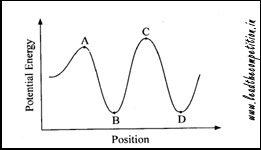### NDA 2012 (2) - Solved Paper

31. Match List I with List II and select the correct answer using the code given below the lists:

List I
(River)
List II
(Sea)
A. Volga 1. Sea of Azov
B. Dnieper 2. Black Sea
C. Rhine 3. Caspian Sea
D. Don 4. Mediterranean Sea

Code:

1. A - 1; B - 4; C - 2; D - 3
2. A - 1; B - 2; C - 4; D - 3
3. A - 3; B - 2; C - 4; D - 1
4. A - 3; B - 4; C - 2; D - 1

32. Which one among the following transitions is associated with the largest change in energy in hydrogen atom?

1. n = 5 to n = 3
2. n = 2 to n = 1
3. n = 3 to n = 2
4. n = 4 to n = 2

33. Match List I with List II and select the correct answer using the code given below the lists:

List I
(Compound)
List II
(Use)
A. Cellulose nitrate 1. Soft soap
B. Potassium sulphate 2. Gun powder
C. Potassium salt of fatty acids 3. Fertilizer
D. Calcium oxide 4. Glass

Code:

1. A - 2; B - 3; C - 1; D - 4
2. A - 2; B - 1; C - 3; D - 4
3. A - 4; B - 1; C - 3; D - 2
4. A - 4; B - 3; C - 1; D - 2

34. Which one among the following does not have an allotrope?

1. Oxygen
2. Sulphur
3. Nitrogen
4. Carbon

35. Match List I with List II and select the correct answer using the code given below the lists:

List I
(Scientist)
List II
(Discovery)
A. Goldstein 1. Atomic theory
C. JJ Thomson 3. Neutron
D. John Dalton 4. Electron

Code:

1. A - 2; B - 3; C - 4; D - 1
2. A - 2; B - 4; C - 3; D - 1
3. A - 1; B - 4; C - 3; D - 2
4. A - 1; B - 3; C - 4; D - 2

36. Consider the following statements:

1. Diamond is hard and graphite is soft.
2. Diamond is soft and graphite is hard.
3. Diamond is a bad conductor but graphite is a good conductor.
4. Diamond is a good conductor but graphite is a bad conductor.

Select the correct answer using the code given below:

Code:

1. 1 and 3
2. 1 only
3. 2 and 3
4. 1 and 4

37. The most important raw materials used in the manufacture of cement are

1. Potassium nitrate, charcoal and sulphur
2. Limestone, clay and gypsum
3. Transition metal oxides, sodium hydroxide or potassium hydroxide
4. Limestone, sodium carbonate and silica

38. Which one among the following equations is correctly balanced?

1. NaOH + Al + H2O --> 2H2 + NaAlO2
2. 2NaOH + 2Al + 2H2O --> 3H2 + 2NaAlO2
3. 2NaOH + 2Al + 3H2O --> 4H2 + 2NaAlO2
4. 2NaOH + 2Al + H2O --> H2 + 2NaAlO2

39. Three elements Na, S and O combine to form a compound Na2SO3. What is the valency of S in this compound?

1. 2+
2. 4+
3. 6+
4. 8+

40. Which one among the following is the equivalent weight of sulphuric acid?

(Atomic weight : H = 1, S = 32, O = 16)

1. 98
2. 60
3. 100
4. 49

41. Deionised water is produced by

1. Calgon process
2. ion-exchange resin process
3. Clark's process
4. Permutit process

42. Which one among the following is a double salt?

1. K4[Fe(CN)6]
2. K2SO4Al2(SO4)324H2O
3. CuSO45H2O
4. NaCl

43. Which one among the following is the correct order of strength of acids?

1. H2SO4 > H3PO3 > CH3COOH
2. H3PO3 > H2SO4 > CH3COOH
3. CH3COOH > H3PO3 > H2SO4
4. CH3COOH > H2SO4 > H3PO3

44. Given below is an approximate composition of a substance:

CaO - 60-70%

SiO2 - 20-25%

Al2O3 - 5-10%

Fe2O3 - 2-3%

The substance is

1. Plaster of Paris
2. Cement
3. Marble stone
4. Quartz

45. In KMnO4 molecule, the oxidation states of the elements Potassium (K), Manganese (Mn) and Oxygen (O) are respectively

1. +1, +5, -2
2. +1, +7, -2
3. 0, 0, 0
4. +1, +7, 0

46. Air is

1. always a compound
2. always a mixture
3. a compound in pollution free zones
4. a mixture in industrial zones

47. Consider the following figure:Which of the following labelled points in the figure given above indicate unstable state of an object?

1. Point A only
2. Point B only
3. Points A and C
4. Points B and D